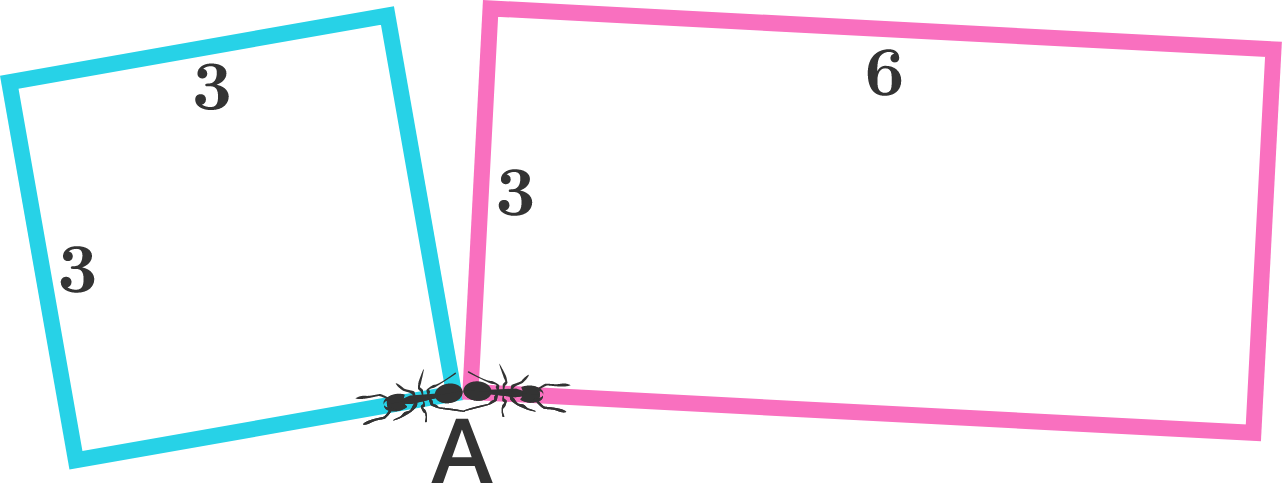The Ants

Geometry Level 1

Two ants start to move from vertex $A$ at the same speed at the same time. One moves along the square with side length $3$, and the other along the rectangle with dimensions $3\times6$. The rectangle and square do not intersect other than at $A$.

Find the minimum distance that each ant must cover before they meet again with each other.×